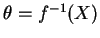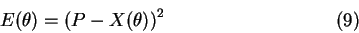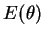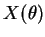Next: Cyclic coordinate descent (CCD) Up: Iterative methods Previous: Iterative model

### Optimization based method

The basic idea of optimization based method is to take a look at the primary equationas minimization problem [Wel93]. Thus, the previous equation could be transformed intoAfter that, standard iterative non-linear optimization techniques could be applied to minimize error function, whereis the goal position of end effector andis the current position of the end effector [PFTV90].

Gradient-based optimization could be also used. This may increase the computation cost in each iteration step, but the convergence rate should be better and the number of iteration steps should decrease.

Lukas Barinka 2002-03-21